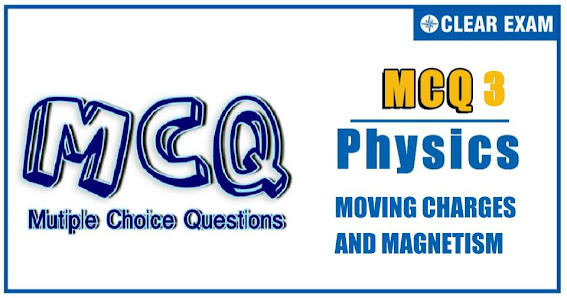## [LATEST]\$type=sticky\$show=home\$rm=0\$va=0\$count=4\$va=0

JEE Advanced Physics Syllabus can be referred by the IIT aspirants to get a detailed list of all topics that are important in cracking the entrance examination. JEE Advanced syllabus for Physics has been designed in such a way that it offers very practical and application-based learning to further make it easier for students to understand every concept or topic by correlating it with day-to-day experiences. In comparison to the other two subjects, the syllabus of JEE Advanced for physics is developed in such a way so as to test the deep understanding and application of concepts.

Q1. Figure shows an equilateral triangle ABC of side l carrying currents as shown, and placed in a uniform magnetic field B perpendicular to the plane of triangle. The magnitude of magnetic force on the triangle is
•  ilB
•  2ilB
•  3ilB
•  zero
Q2.An electron accelerated through a potential difference V passes through a uniform transverse magnetic field and experience a force F. If the accelerating potential is increased to 2V, the electron in the same magnetic field will experience a force
•  F
•  F/2
•  √2 F
•  2F
Q3.  Figure shows a conducting loop ABCDA placed in a uniform magnetic field (strengthB) perpendicular to its plane. The part ABC is the (3⁄4)^th portion of the square of side length l. The part ADC is a circular arc of radiusR. The points A and C are connected to a battery which supplies a current I to the circuit. The magnetic force on the loop due to the field B is
•  zero
•  BIl
•  2 𝐵𝐼𝑅
•  BIlR/(I+R)
Solution

Q4. Ion a moving coil galvanometer, the deflection of the coil θ is related to the electric current i by the relation
•  i∝tan⁡θ
•  i∝θ
•  i∝θ^2
•  i∝√θ
Solution
Q5. A Q5.A particle with a specific charge s is fired with a speed v toward a wall at a distance d, perpendicular to the wall. What minimum magnetic field must exist in this region for the particle not to hit the wall?

•  v/sd
•  2v/sd
•  v/2 sd
•  v/4 sd
Q6. A particle of charge q and mass m starts moving from the origin under the action of an electric field E ⃗=E_0 i ̂ and B ⃗=B_0 i ̂ with a velocity v ⃗=v_0 j ̂. The speed of the particle will become 2v_0 after a time

•  t=(2mv_0)/qE
•  t=2Bq/(mv_0 )
• t=(√3 Bq)/(mv_0 )
•  t=(√3 mv_0)/qE
Q7.The force between two parallel current carrying wires is independent of

•  Their distance of separation
•  The length of the wires
•  The magnitude of currents
•  The radii of the wires
Q8.A proton of mass 1.67×10^(-27) kg and charge 1.67×10^(-19) C is projected with a speed of 2×10^6 ms^(-1) at an angle of 60° to the X-axis. If a uniform magnitude field of 0.10 T is applied along Y-axis, the path of proton is
•  A circle of radius 0.2 m and time period π×10^(-7)s
•  TA circle of radius 0.1 m and time period 2π×10^(-7)s
•  A helix of radius 0.1 m and time period 2π×10^(-7)s
•  A helix of radius 0.2 m and time period 4π×10^(-7)s
Q9.An equilateral triangular loop is kept near to a current carrying long wire as shown in figure under the action of magnetic force alone, the loop
•  Must move along positive or negative X-axis
•  Must move in XY plane and not along X-or Y-axis
•  Does not move
•  Moves but which way we cannot predict
Q10. Two infinite long wires, each carrying current I, are lying along x-and y-axis, respectively. A charged particle, having a charge q and mass m, is projected with a velocity u along the straight line OP.. the path of the particle is (neglect gravity)
•  Straight line
•  Circle
•  Helix
• Cycloid
Solution#### Written by: AUTHORNAME

AUTHORDESCRIPTION## Want to know more

Please fill in the details below:

## Latest NEET Articles\$type=three\$c=3\$author=hide\$comment=hide\$rm=hide\$date=hide\$snippet=hide

Name

ltr
item
BEST NEET COACHING CENTER | BEST IIT JEE COACHING INSTITUTE | BEST NEET, IIT JEE COACHING INSTITUTE: Moving Charges and Magnetism-Quiz-3
Moving Charges and Magnetism-Quiz-3
https://1.bp.blogspot.com/-nfc-ecZRU3A/YOXZkDn8rmI/AAAAAAAAAF0/WO7BsTocM-wiAeOWGSWXyEdi64a_aLP9QCLcBGAsYHQ/w567-h318/Quiz%2BImage%2B20.jpg
https://1.bp.blogspot.com/-nfc-ecZRU3A/YOXZkDn8rmI/AAAAAAAAAF0/WO7BsTocM-wiAeOWGSWXyEdi64a_aLP9QCLcBGAsYHQ/s72-w567-c-h318/Quiz%2BImage%2B20.jpg
BEST NEET COACHING CENTER | BEST IIT JEE COACHING INSTITUTE | BEST NEET, IIT JEE COACHING INSTITUTE
https://www.cleariitmedical.com/2021/07/moving-charges-and-magnetism-quiz-3.html
https://www.cleariitmedical.com/
https://www.cleariitmedical.com/
https://www.cleariitmedical.com/2021/07/moving-charges-and-magnetism-quiz-3.html
true
7783647550433378923
UTF-8